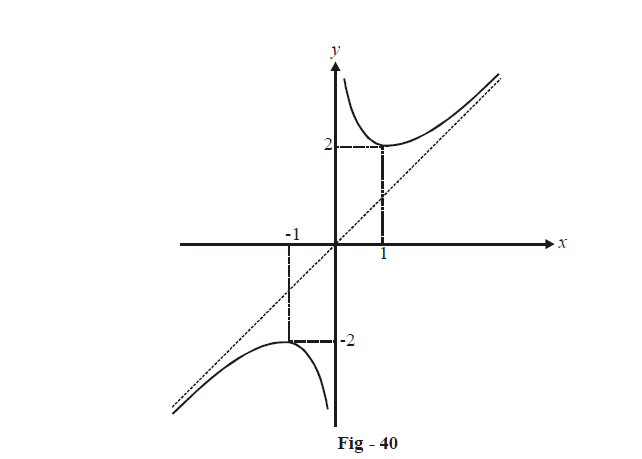# Asymptotes in Graphs

Go back to  'Applications of Derivatives'

Learn from the best math teachers and top your exams

• Live one on one classroom and doubt clearing
• Practice worksheets in and after class for conceptual clarity
• Personalized curriculum to keep up with school

## Asymptotes

A straight line is called an asymptote to the curve y = f (x) if, in layman’s term, the curve touches the line at infinity (this is not technically correct; we should say that the curve tends to touch the line as infinity is approached or as $$x \to \infty$$)

More accurately, an asymptote to a curve is a line such that the distance from a variable point M on the curve to the straight line approaches zero as the point M recedes to infinity along some branch of the curve.

Referring to the previous example, we see that y = 0 is a horizontal asymptote to \begin{align}f\left( x \right) = \frac{x}{{1 + {x^2}}};\end{align} x = 1 and x = 2 are vertical asymptotes to \begin{align}f\left( x \right) = \frac{1}{{{x^2} - 3x + 2}}\end{align} and y = 1 is a horizontal asymptote to \begin{align}f\left( x \right) = \frac{{{x^2} - x + 1}}{{{x^2} + x + 1}}\end{align}. Of course, there can be inclined asymptotes also. We now formally distinguish between the three kinds of asymptotes and outline the approach to determine them.

(a) Horizontal asymptotes:  If $$\mathop {\lim }\limits_{x \to \pm \infty } f\left( x \right) = k$$ then y = k is a horizontal asymptote to f (x).

(b) Vertical asymptotes:       If LHL or RHL (or both) at x = a are infinity for f (x), then

x = a  is a vertical asymptote to f (x).

(c) Inclined asymptotes:      If and \begin{align}\mathop {\lim }\limits_{x \to \infty } \frac{{f\left( x \right)}}{x} = {a_1}\end{align}, then \begin{align}y={{a}_{1}}x+~{{b}_{1~}}\end{align} is an inclined right asymptote to f (x).

Similarly, if \begin{align}\mathop {\lim }\limits_{x \to - \infty } \frac{{f\left( x \right)}}{x} = {a_2}\end{align} and $$\mathop {\lim }\limits_{x \to \infty } \left( {f\left( x \right) - {a_1}x} \right) = {b_1}$$, then $$y = {a_2}x + {b_2}$$ is an inclined left asymptote to f(x).

This discussion will become more clear with an example.

Let                                                  \begin{align}f\left( x \right) = x + \frac{1}{x}\end{align}

Now,                                               $$\mathop {\lim }\limits_{x \to {0^ + }} f\left( x \right) = \infty \,\,\,{\rm{and}}\,\,\mathop {\lim }\limits_{x \to {0^ - }} f\left( x \right) = - \infty$$

$$\Rightarrow \,\,\, x = 0$$ is a vertical asymptote to $$f\left( x \right)$$.

\begin{align}\mathop {\lim }\limits_{x \to \infty } \frac{{f\left( x \right)}}{x} = 1; \mathop {\lim }\limits_{x \to - \infty } \frac{{f\left( x \right)}}{x} = 1\end{align}

$$\mathop {\lim }\limits_{x \to \pm \infty } \left( {f\left( x \right) - x} \right) = 0.\,\,\,\left( {\because a = 1,\,b = 0} \right)$$

$$\Rightarrow \quad y = x$$ is an inclined asymptote to f(x).

The graph is sketched below. The extremum points are $$x = \pm 1:$$Before closing this section with a few more examples, have are a few general steps* to be followed whenever one is encountered with the task of sketching the graph of an arbitrary function f (x):

(i)       Find the domain of the given function.

(ii)      Determine more of its characteristics; for example, is the function even or odd or neither? Is it periodic? If yes, what is the period? And so on.

(iii)     Test the function for continuity and differentiability.

(iv)     Find the asymptotes of the graph, if any.

(v)      Find the extremum/ inflexion points and the intervals of monotonicity.

(vi)      To improve accuracy of the plot, one can always evaluate f (x) at additional points.

This is a very general sequence and mostly the graph would be able to be plotted without necessarily following all the steps. For all our current purposes, these steps are more than sufficient.

Applications of Derivatives
grade 11 | Questions Set 1
Applications of Derivatives
Applications of Derivatives
grade 11 | Questions Set 2
Applications of Derivatives